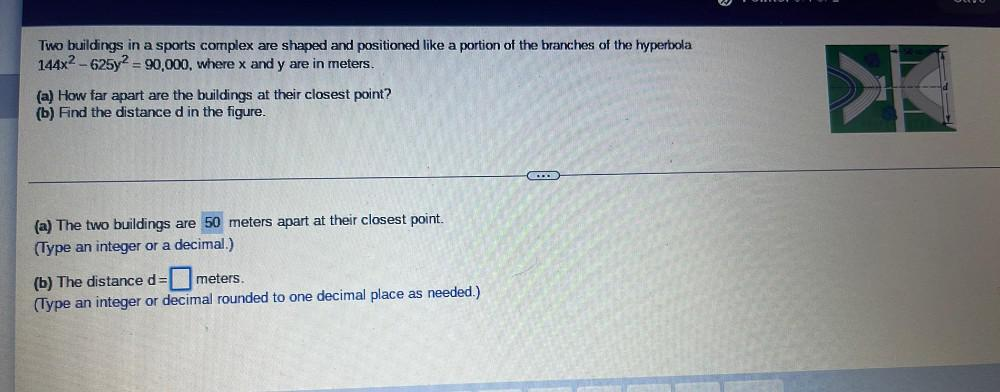Question:

# Two buildings in a sports complex are shaped and positioned like a portion of the branches of the hyperbola 144x² - 625y² = 90,000, where x and y are in meters. (a) How far apart are the buildings atTwo buildings in a sports complex are shaped and positioned like a portion of the branches of the hyperbola 144x² - 625y² = 90,000, where x and y are in meters. (a) How far apart are the buildings at their closest point? (b) Find the distance d in the figure. (a) The two buildings are 50 meters apart at their closest point. (Type an integer or a decimal.) (b) The distance d= ▢ meters. (Type an integer or decimal rounded to one decimal place as needed.)